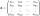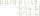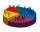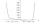# The determinant

The determinant of the unit matrix equals 7. Check how many rows the A matrix contains.

Result

n =  7

#### Solution:Leave us a comment of example and its solution (i.e. if it is still somewhat unclear...):Be the first to comment!## Next similar examples:

1. Inverse matrixFind how many times is the larger determinant is the matrix A, which equals 9 as the determinant of its inverse matrix.
2. The inverseThe inverse matrix for matrix A has a determinant value of 0.333. What value has a determinant of the matrix A?
3. Inverse matrixFind out inverse by Gauss elimination or by reduction method. A=[2/3. 1 -3. 1/3]
4. EquationEquation ? has one root x1 = 8. Determine the coefficient b and the second root x2.
5. RootsDetermine the quadratic equation absolute coefficient q, that the equation has a real double root and the root x calculate: ?
6. DiscriminantDetermine the discriminant of the equation: ?
7. BallsWe have n identical balls (numbered 1-n) is selected without replacement. Determine 1) The probability that at least one tensile strength number coincides with the number of balls? 2) Determine the mean and variance of the number of balls, which coincides.
8. AS sequenceIn an arithmetic sequence is given the difference d = -3 and a71 = 455. a) Determine the value of a62 b) Determine the sum of 71 members.
9. SeatsSeats in the sport hall are organized so that each subsequent row has five more seats. First has 10 seats. How many seats are: a) in the eighth row b) in the eighteenth row
10. Fish tankA fish tank at a pet store has 8 zebra fish. In how many different ways can George choose 2 zebra fish to buy?
11. Unknown numberI think number. If subtract from the twelfth square the ninth square I get a number 27 times greater than the intended number. What is this unknown number?
12. Theorem proveWe want to prove the sentence: If the natural number n is divisible by six, then n is divisible by three. From what assumption we started?
13. Geometric progression 2There is geometric sequence with a1=5.7 and quotient q=-2.5. Calculate a17.
14. SequenceBetween numbers 1 and 53 insert n members of the arithmetic sequence that its sum is 702.
15. Euclidean distanceCalculate the Euclidean distance between shops A, B and C, where: A 45 0.05 B 60 0.05 C 52 0.09 Wherein the first figure is the weight in grams of bread and second figure is price in USD.
16. Sequence 3Write the first 5 members of an arithmetic sequence: a4=-35, a11=-105.
17. Complex number coordinatesWhich coordinates show the location of -2+3i